Printed from www.flong.com/texts/code/shapers_bez/
Contents © 2019 Golan Levin and Collaborators

# Golan Levin and Collaborators

## Bezier and Other Parametric Shaping Functions

• Quadratic Bezier
• Cubic Bezier
• Cubic Bezier (Nearly) Through Two Given Points

Quadratic Bezier

This function defines a 2nd-order (quadratic) Bezier curve with a single user-specified spline control point (at the coordinate a,b) in the unit square. This function is guaranteed to have the same entering and exiting slopes as the Double-Linear Interpolator. Put another way, this curve allows the user to precisely specify its rate of change at its endpoints in the unit square.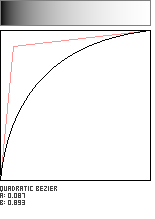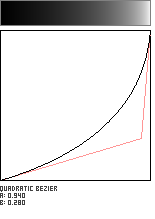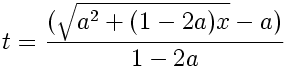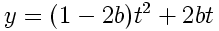```//------------------------------------------------
float quadraticBezier (float x, float a, float b){
// adapted from BEZMATH.PS (1993)
// by Don Lancaster, SYNERGETICS Inc.
// http://www.tinaja.com/text/bezmath.html

float epsilon = 0.00001;
a = max(0, min(1, a));
b = max(0, min(1, b));
if (a == 0.5){
a += epsilon;
}

// solve t from x (an inverse operation)
float om2a = 1 - 2*a;
float t = (sqrt(a*a + om2a*x) - a)/om2a;
float y = (1-2*b)*(t*t) + (2*b)*t;
return y;
}
```

Cubic Bezier

The Cubic Bezier is a workhorse of computer graphics; most designers will recognize it from Adobe Illustrator and other popular vector-based drawing programs. Here, this extremely flexible curve is used in as a signal-shaping function, which requires the user to specify two locations in the unit square (at the coordinates a,b and c,d) as its control points. By setting the two control points (a,b) and (c,d) to various locations, the Bezier curve can be used to produce sigmoids, seat-shaped functions, ease-ins and ease-outs.

Bezier curves are customarily defined in such a way that y and x are both functions of another variable t. In order to obtain y as a function of x, it is necessary to first solve for t using successive approximation, making the code longer than one might first expect. The implementation here is adapted from the Bezmath Postscript library by Don Lancaster.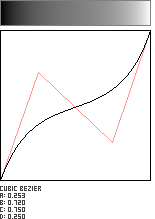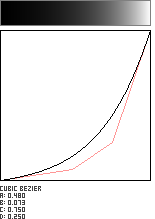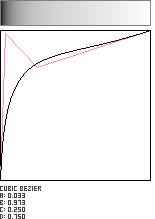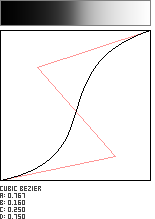```//--------------------------------------------------------------
float cubicBezier (float x, float a, float b, float c, float d){

float y0a = 0.00; // initial y
float x0a = 0.00; // initial x
float y1a = b;    // 1st influence y
float x1a = a;    // 1st influence x
float y2a = d;    // 2nd influence y
float x2a = c;    // 2nd influence x
float y3a = 1.00; // final y
float x3a = 1.00; // final x

float A =   x3a - 3*x2a + 3*x1a - x0a;
float B = 3*x2a - 6*x1a + 3*x0a;
float C = 3*x1a - 3*x0a;
float D =   x0a;

float E =   y3a - 3*y2a + 3*y1a - y0a;
float F = 3*y2a - 6*y1a + 3*y0a;
float G = 3*y1a - 3*y0a;
float H =   y0a;

// Solve for t given x (using Newton-Raphelson), then solve for y given t.
// Assume for the first guess that t = x.
float currentt = x;
int nRefinementIterations = 5;
for (int i=0; i < nRefinementIterations; i++){
float currentx = xFromT (currentt, A,B,C,D);
float currentslope = slopeFromT (currentt, A,B,C);
currentt -= (currentx - x)*(currentslope);
currentt = constrain(currentt, 0,1);
}

float y = yFromT (currentt,  E,F,G,H);
return y;
}

// Helper functions:
float slopeFromT (float t, float A, float B, float C){
float dtdx = 1.0/(3.0*A*t*t + 2.0*B*t + C);
return dtdx;
}

float xFromT (float t, float A, float B, float C, float D){
float x = A*(t*t*t) + B*(t*t) + C*t + D;
return x;
}

float yFromT (float t, float E, float F, float G, float H){
float y = E*(t*t*t) + F*(t*t) + G*t + H;
return y;
}
```

Cubic Bezier (Nearly) Through Two Given Points

This shaping function asks the user to specify two points in the unit square. The algorithm then attempts to generate a curve which passes through these points as closely as possible. The curves are not guaranteed to pass through the two points, but come quite close in most instances. The method is adapted from Don Lancaster.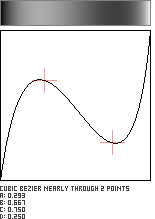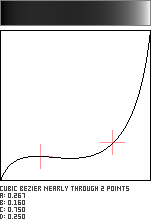```//--------------------------------------
float cubicBezierNearlyThroughTwoPoints(
float x, float a, float b, float c, float d){

float y = 0;
float epsilon = 0.00001;
float min_param_a = 0.0 + epsilon;
float max_param_a = 1.0 - epsilon;
float min_param_b = 0.0 + epsilon;
float max_param_b = 1.0 - epsilon;
a = max(min_param_a, min(max_param_a, a));
b = max(min_param_b, min(max_param_b, b));

float x0 = 0;
float y0 = 0;
float x4 = a;
float y4 = b;
float x5 = c;
float y5 = d;
float x3 = 1;
float y3 = 1;
float x1,y1,x2,y2; // to be solved.

// arbitrary but reasonable
// t-values for interior control points
float t1 = 0.3;
float t2 = 0.7;

float B0t1 = B0(t1);
float B1t1 = B1(t1);
float B2t1 = B2(t1);
float B3t1 = B3(t1);
float B0t2 = B0(t2);
float B1t2 = B1(t2);
float B2t2 = B2(t2);
float B3t2 = B3(t2);

float ccx = x4 - x0*B0t1 - x3*B3t1;
float ccy = y4 - y0*B0t1 - y3*B3t1;
float ffx = x5 - x0*B0t2 - x3*B3t2;
float ffy = y5 - y0*B0t2 - y3*B3t2;

x2 = (ccx - (ffx*B1t1)/B1t2) / (B2t1 - (B1t1*B2t2)/B1t2);
y2 = (ccy - (ffy*B1t1)/B1t2) / (B2t1 - (B1t1*B2t2)/B1t2);
x1 = (ccx - x2*B2t1) / B1t1;
y1 = (ccy - y2*B2t1) / B1t1;

x1 = max(0+epsilon, min(1-epsilon, x1));
x2 = max(0+epsilon, min(1-epsilon, x2));

// Note that this function also requires cubicBezier()!
y = cubicBezier (x, x1,y1, x2,y2);
y = max(0, min(1, y));
return y;
}

// Helper functions.
float B0 (float t){
return (1-t)*(1-t)*(1-t);
}
float B1 (float t){
return  3*t* (1-t)*(1-t);
}
float B2 (float t){
return 3*t*t* (1-t);
}
float B3 (float t){
return t*t*t;
}
float  findx (float t, float x0, float x1, float x2, float x3){
return x0*B0(t) + x1*B1(t) + x2*B2(t) + x3*B3(t);
}
float  findy (float t, float y0, float y1, float y2, float y3){
return y0*B0(t) + y1*B1(t) + y2*B2(t) + y3*B3(t);
}
```